GEOMETRY FUN

2 Dimensional Geometry

In this essay we’re going to have a little fun pretending that we’re the creator of the universe. In essence, we're going to play God. If you're religious and are offended by this idea then I'm sorry, but if you're like me, you've sometimes wondered what the world would be like if you got to make all the decisions. So, in a spirit of good fun, let's continue.
Let’s say there is nothing in the universe, because nothing has been created yet. If nothing existed at all, and there were no galaxies, stars, planets, or even atoms, how would we begin the creative process?
Well, even though I'm playing God, I'm really just a dopey human, so we'll stick with something simple: building some simple 2 dimensional polygons. (Poly = many, gon = side).

We’ll say that, like in dreams, our thoughts can manifest instantly. We’ll also say that we don’t know anything to start off; in other words, our consciousness is like a newborn baby and we don’t have any idea of physics or chemistry or what a planet looks like, or what atoms and molecules are, because they haven’t been thought up yet! We’ll say that each thought we have creates a little point mass, and that we can combine them together.
So right now we have a consciousness that is just hanging out, and it's really boring because there's nothing to do! Nothing to play around with.
Let’s call our self-aware consciousness Hal (actually consciousness doesn’t have gender, but we’ll say Hal is a him). Before we get started, we'll leave Hal hanging out in space for a bit and look at a few assumptions; after all, he'll need plenty of time to think about how he's going to proceed.
If you want you can just skip this part and go right to Let’s Get Started below.

Here are the assumptions we’ll be operating under:

1) The fundamental 'particle' or quanta of energy in the universe is a thought. Let’s call this a POINT.
2) All matter and energy will be ultimately composed of POINTS.
3) We’ll say that POINT’s are conscious, because they are thoughts, and since we are conscious, so are our thoughts. In other words, POINTS  are conscious, because they proceed directly from consciousness.
4) POINT’s can perceive  omni-directionally. Meaning, that if you placed your awareness in a POINT, you can perceive in all directions.
Euclid defined a POINT as, “That which has no part.” We’re saying here that a POINT is not an abstraction, it has consciousness and existence. In other words, for our little game, we’ll say a POINT is real.

We will say that a LINE is  the connection of awareness between 2 POINTS. It comes about when we create another POINT, or, when two POINTS become aware of each other.
A LINE is either curved or straight. If it is perfectly straight then there is instant communication, because there is absolutely no change of direction. So there is no time between a communication from one end and the receipt of that communication at the other. We’ll say there’s no medium, like water, or air, or “ether,” to stop our perception. We’ll assume that each POINT has a sort of instantaneous telepathy with other POINTS, and with us.
If the LINE is curved then there is a point within the concave boundary of the line from which all the other points are generated and to which they are all related. The arc of a circle is an example of a curved line. The center of the circle is the point to which all of the other points on the circumference are related (all the points on the circumference are the same distance from the center).
Because curved lines are constantly changing direction, there is persistence. In other words, to go in a new direction  requires a decision at every step along the way, a slight hesitancy which creates time.

A note on central angles: a central angle is the angle a point on the circumference makes with the center of the circle and an adjacent point on the circumference. If you look at the diagrams below, you will see that the central angle is also a measure of the chord length of the polygon (the side of the polygon).

Assertions:
All polygons are inscribed in circles. The circle is the beginning point for ALL 2  dimensional geometry.
The circle is  Unity.
Growth comes from the division of Unity.
The circle provides the reference point from which geometric order proceeds. In other words, all Form  is created from Unity.

Let’s Get Started!

To follow along with this discussion, you can get out paper, a straight edge, and a compass.

OK, Hal is hanging out in nothingness (pretend that you are Hal). There isn’t even any space yet, just a pure potential who is self-aware. How does he start to make anything?
Well, the first thing Hal decides is that it would be cool to have something outside of himself to look at.  As soon as Hal decides that, he has just created a POINT! Lets call that POINT A. Hal has also created space between himself and A, so he can view it.
In other words, without space, there would be no separation between Hal and POINT A. Hal now realizes that POINT A is different from him, because it occupies a different point in space. At the same time, he realizes that POINT A is his thought, so Hal can identify with A as well. This is a state of affairs Hal has never considered before, because prior to the creation of A, there was just Hal and nothing else.
A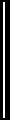O
OA = r

After pondering this for a while, Hal realizes he has a radius, or a limiting point of perception.  Hal is at O and has just created a LINE OA. What can he do with that?
Well, right now the only distance Hal knows is r, but he gets the brilliant idea of making more and more POINTS the same distance away. And when he does that, he is astonished to have created a circle. Hal can see right off the bat that once the circle is connected, it really has no beginning or ending, and it reminds Hal of himself, for he can never remember a time when he was not self aware.
All of the points Hal will create, up to and including length r, will be his universe. He can have a whole bunch of POINTs, each of which is conscious, the outer boundary of which represents the  circumference of the circle of his awareness (we are only talking 2 dimensions here). For our purposes we will deal only with points on the circumference.

A circle – Hal’s universe.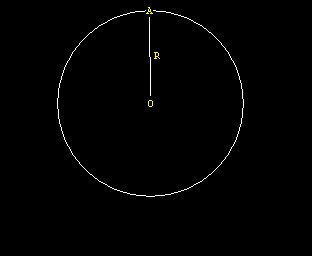What’s next?
The only information Hal has is r. He has created a bunch of connected POINTS to form a circle, but what can he do with that?
Hal decides to go to A and look through A at his circle. He admires his circle and thinks that it is really the most wonderful thing in the universe. And since it is the only thing in the universe at this juncture, Hal is perfectly correct!
Hal gets the brilliant idea to go to another POINT the same distance r from A on his circle, and he calls that POINT B. Hal has no idea what’s going to happen with this, but he’s really excited so he looks in the other direction from A and moves to another POINT the same distance, r, at C. At C he does the same thing to get to D, and at D to create E.
Now Hal realizes something really cool:  the 6 points, A,B,C,D,E,F are all exactly spaced around the circumference.

If you are doing this with compass straight-edge and paper, start by pinning the compass at A, with length OA, and find the intersection with the  circumference at B  and C. Pin the compass  at B and find another point of intersection with the circumference at D . Pin the compass at C and find another point of intersection at E. From E (or  D)  find F.

The hexagon -- the first regular polygon in Hal’s universe. Central angle (AOB) 360 / 6 = 60 degrees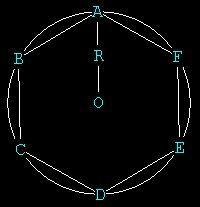Another way to get the hexagon is pin the compass at A and draw a circle, then pin the compass at D and draw another circle. The intersections of the three circles will form the POINTs of the hexagon: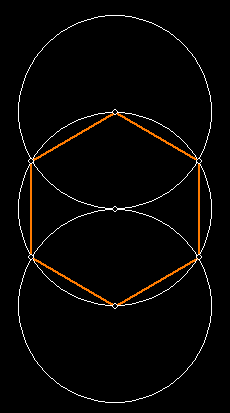We have divided the circle into 6 parts and have identified 6 POINTs and 6 LINEs.
The LINES in the diagram represent the communication between each conscious POINT in Hal’s hexagon. Because the POINTS A,B,C,D,E  have been created by Hal, they have a little bit of Hal’s conscious awareness 'built in' to them, as an inherent part of their nature. They can be used by Hal as positions from which to view the hexagon from any POINT.
When Hal places his awareness at any POINT he sees the exact same thing, because all of the distances are equal around the circumference and to the center.

We are now at F.   From F with the straight-edge connect to B and C. We have now created our second regular polygon, the equilateral triangle.
The triangle is the minimum polygon, with 3 vertices.
From A connect to D and E. Now we have, inscribed in the circle, 2 interlocking equilateral triangles. When we look at this figure 3 dimensionally later, it will be 2 interlocking tetrahedron's forming the vertices of a cube.

The circumscribed Equilateral Triangle -- the minimum  polygon in our universe
Central angle (AOE)   360 / 3 = 120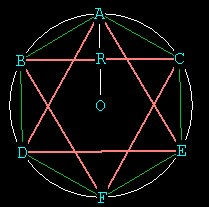The first regular polygon in creation, then is the hexagon! This seems counter intuitive, doesn't it? How could something with 6 vertices come before something with 3 vertices? Of course you can stop after the first 3 points A, B, C and connect them to form a triangle, but this triangle doesn't have equal sides. Remember, all we knew going around the circle distance-wise was r, the radius!
Hal could  have connected immediately to O after making B and formed an equilateral triangle, but this would involve him as one of the vertices.
So in the beginning, there is a circle, and then 6 points on the circle forming the hexagon. From the 6, immediately comes the 3.
As we gaze out upon our hexagon and our triangles we realize that the lines of communication to all 6 points form 6 smaller equilateral triangles all with side lengths equal to r. We realize that the triangle is the simplest polygon and that 6 equilateral triangles could form a hexagon. We also can see that the intersections of the lines of the equilateral triangles forms another hexagon inside, and that this interior hexagon is rotated 30 degrees from the outer one.

We are on a roll now. We have now created a  regular polygon with 6 vertices and one with 3. Lets try and see if we can construct one with 4 vertices.  After  a little thought we figure it out. First with your straight-edge connect up points A and F. That's the easy part. We now have the 'y axis.' To get the 'x axis' we have to figure out how to bisect the lines BD and CE.
We still have our compass set to r! Pin it at B and draw and arc above BD. Pin it at D and draw an arc to intersect the first one. Do the same  with the line  CE. Now with the straight-edge connect the two points together, going through O.
The intersection of this line with the circle comes at H and at I. The line IH is called a perpendicular bisector of the line AF, through O. Now IH is our 'x axis.'

Bisecting the line   xx'---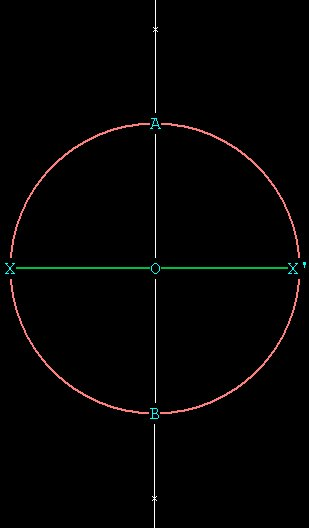Pin the compass at x with the other leg at x', and mark an arc above and below the circle.
Pin the compass at x' with the other leg at x, and mark an arc above and below the circle that intersects with the other arc.  Now connect the line through O.

Now connect A, I, F, H to form the square. We can see that these points are regularly spaced because the angles between them are equal.

The Square --  Central angle(AOH) = 360 / 4  = 90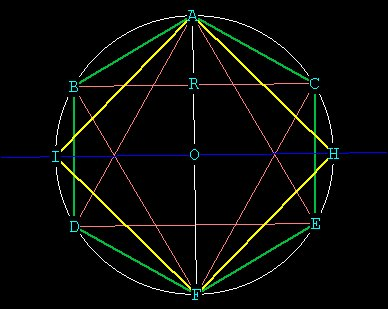We have created 2 more POINTs I and H and now have 4 polygons, a hexagon (green), 2 equilateral triangles, (pink), and a square (yellow). Here we see that growth comes from further division into Unity.
Now that we have our square, we can proceed to the 8 sided polygon, or octagon.
All we have to do is bisect each one of the sides of the square, which is the same thing as bisecting the central angles of the square.  We bisect lines AI and FH through O, and bisect lines AH and IF through O, to get points L,M,N, K

The octagon -- formed from the square (the octagon is in red). Central angle (MOH) 360/8 = 45Now lets try for the 5-sided polygon to complete sequence of 3,4,5,6.
This one is a little tricky and requires a lot of thought. See "The Construction of the Pentagon" for instructions.

The Pentagon ---  representing continuous harmonic growth. Central angle (GOE) = 360/5 = 72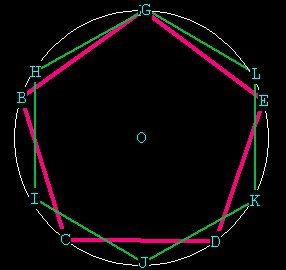From the pentagon we can immediately get the 10-gon, or decagon, by bisecting the sides (and angles) of the pentagon, just like we bisected the sides of the square to get the octagon. It turns out that the sides of the decagon are in Phi ratio to the sides of the pentagon!

The decagon --   formed from the pentagon. Central angle VOU = 360 / 10 = 36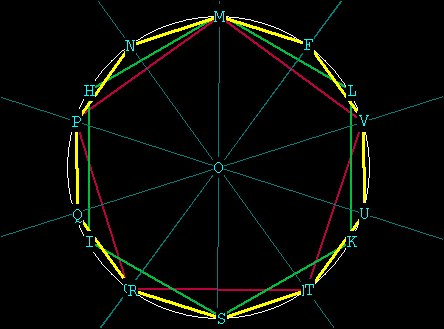Here we have demonstrated all of the polygons from 3 to 10 that can be precisely generated in one and only one circle. There are 2 polygons  which are currently thought to be accurately impossible to draw geometrically:  the 7-gon (heptagon) and the 9-gon (enneagon). The 7-gon is impossible to construct because the central angles which represent its edges are repeating decimals
(i.e. 360 / 7 = 51.428571428571..... this number can't be pinned down).
The 9-gon can't be constructed, even though its central angle is an integer (360 / 9 = 40) because it involves the trisection of a 60 degree angle. Think about it.  We need a 40 central angle. We don't have protractors with nice little angles marked off on them, only compass and straight-edge.
So we have to work with an already-defined central angle. We have the following angles from the diagrams shown above:
120, 90, 72, 60, 45, 36.
The problem:   How to get 40 from any of these?
The only possible way is to trisect either the 120 (120 / 3 = 40) or
trisect the 60 (60 / 3 = 20. 20 + 20 = 40). But with the standard compass and straight-edge, we can only bisect!!  If you don't believe it is possible to trisect an angle, get out your paper, compass and straight-edge and try it for yourself!
It turns out we can only construct polygons of 2,4,5,6,8,10,12,15,16, 17,20 sides if we use standard compass and straight-edge. (1)

However, the 7-gon and the 9-gon, in fact ANY polygon can be constructed using the new compass developed by James D. Watt called the Z-wheel.
The Z-wheel  is attached to the marking leg of a standard compass and is used to measure arc lengths.
In footnote (2) below  is a picture of Mr. Watt's compass, taken from his website, with a brief description.
With this compass any n-gon can be constructed by the multiplication of radial distances. In this method, PI is unnecessary. The unit of measure is simply the linear, whole number, radial distance which automatically generates a circumference. The only difficulty with this method is that it requires, in particular cases, n concentric circles to produce an n-sided polygon. But that doesn't detract from its power.

Of course, some people will say this is cheating, for it is really impossible to find and mark an exact point on the z-wheel, but there is also an error when pinning a standard compass to any point! The z-wheel is an interesting idea because it uses 3 dimensions to solve a puzzle in 2 dimensions. And anyway, we're just having fun, so we can do whatever we want!

First lets start with the 9-gon, go to the 7-gon and then show and by that time it will be obvious that the method can be applied to any n-gon.

With the Z-wheel compass arc lengths can  be measured and angles can  be trisected. The trisection depends upon making a circle which has a radius 3 times the length of our original circle. The z-wheel is then rotated around the first circle from point A to point B and the arc distance noted from the wheel.. Then that same distance is marked 3 times upon the circle whose circumference is 3 times a large as the original circle, and the angle is trisected. (See Mr. Watt's geometry pages for a thorough and much more precise explanation of the compass, as well as an overhauling of Euclidian geometry, at http://www.compasswatt.com).

The 9-gon (in light green) -- formed by multiplying  the radius of the original circle by 3.
Central angle (AGH) 360 / 9 = 40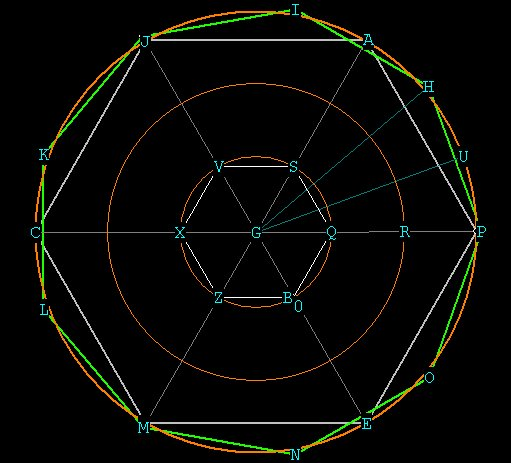Our original circle is the inner circle with radius GQ.  GQ is considered to be Unity with respect to the other radial distances.  We have divided into the circle with radius GQ to form the hexagon marked in white, which we shall use as a reference as we expand outward.  Place the point of the compass at Q, to get R, and draw the circle with radius GR. Pin the compass at R to get P, and draw the circle with radius GP. Walk the compass around circle GP to get the hexagon marked in gray. We now have 3 circles such that the radial distance of GR is exactly twice that of GQ, and the radial distance GP is precisely three times that of GQ.
The central angles of the hexagon (for example, <AGP) are all 60 degrees (360 / 6 points of the heaxagon ).  In order to get the 9-gon, we have to come up with a 40 degree angle. The best way to do that is to work with the hexagon, and cut the <AGP in thirds.
Take the z-wheel and pin it at G, with the other leg at Q. Then roll the z-wheel to S and note the distance on the wheel. With the compass still pinned at G, set the other leg now to P. Roll off the exact distance you marked on the inner circle (QS) from P on the outer circle until you hit U on the outer circle. Do the same from U to H and from H to A. Now you have cut <AGP in thirds and you have <PGU = <PGH = <PGA = 20 degrees.
So <PGH = 40 degrees, which is the central angle of the 9-gon (360 / 9 = 40). So the first two points on the 9- gon  are P and H. Now you can walk either the standard compass or the Z-wheel compass around the circle to get all of the other points.

Now lets take on the 7-gon (heptagon). Here is a pic of it: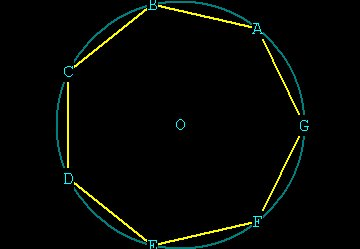Normally we draw our circle and create the polygon inside of it. Using the z-wheel compass, we must use increasing radial distances. here is how it is done: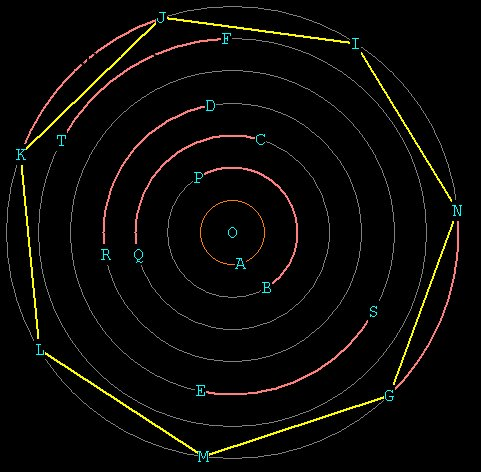We will call the original circle A. We use it's radial distance OA as Unity, from which to derive all of the other distances.  As before, we use the compass to get all of the other circles, which now have radii in integer multiples of the original circle  OA.
We need to get 7 EXACTLY spaced points on the circumference of one of the circles in order to precisely generate the heptagon.
Firstly, note that the circumference of a circle is directly proportional to the radius of a circle:
C = 2PI*r. If the radius increases by whole number increments, then the circumference also increases by that same amount: Lets compare  the circle with radial distance OB, which has radius twice that of Unity, (so OB =  2),  to  circle OF with radius 6:
C(F)  =      __2*PI*6__ =  3. So C(F) = 3* C(B) if the radius is increased 3 times.
C(B) =          2*PI*2

Take the z-wheel compass and pin it at O. Start at A and make 1 complete revolution of the compass wheel until you get back to A again. Note the position on the wheel. We now have, logically, an exact distance for the circumference of circle A. Now all we need to do is go out to circle G, with the compass still pinned at O, and rotate the z-wheel 7 times with the exact arc-distance noted on the wheel to find our 7 points.
I have noted in orange how the exact circumference of circle A is marked off on all of the other circles:  the circumferential distance of circle A is exactly one half that of circle B,  exactly one third that of circle C, etc.
This ingenious solution does not require the use of PI or even messy central angles, it is dependent upon whole number radial distances. The wheel itself contains PI built right into it!

You can see that ANY n-gon can be constructed using this method: at worst it will take n concentric circles to draw any n-gon.
In the 9-gon we only needed 3 circles, because the 60 degree angle on circle A marks out a circumferential distance  only 1/3 the distance on circle C.

Now we come to one of the most talked about problems in 2 dimensional geometry, squaring the circle. Again, this is impossible with the standard compass but not with the Z-wheel compass. By simply tracing the arc length along the wheel and noting how many revolutions, one can simply detach the measuring leg and trace out the linear distance from the Z-wheel in a straight line.  Two bisections of this distance give the length of each side of the square.

______________________________________________________________
Appendix and credits:

(1) paraphrased from Dr. Wilkinson at the Math Forum:
The great mathematician Carl Gauss discovered that a polygon with n sides can only be constructed if n is a power of 2, and if n is a  prime number of the form 2^2^k + 1, where k is a positive integer.
for k = 0,1,2,3,4  we get 3,5,17,257,65537. For 2^k where k = 2,3,4 we get 4,8,16.
Looking just at the numbers from 3 to 20, the following table can be shown
of which regular polygons can and can't be constructed:
3    yes    3 = 2^2^0 + 1
4    yes    4 = 2^2
5    yes    5 = 2^2^1 + 1
6    yes    6 = 2 * 3
7    no
8    yes    8 = 2^3
9    no
10    yes    10 = 2 * 5
11    no
12    yes    12 = 2^2 * 3
13    no
14    no
15    yes    15 = 3 * 5
16    yes    16 = 2^4
17    yes    17 = 2^2^2 + 1
18    no
19    no
20    yes    20 = 2^2 * 5

(2) From the paper called "Repairing a Deficiency in Euclidian Geometry" by James Watt available at www.compasswatt.com: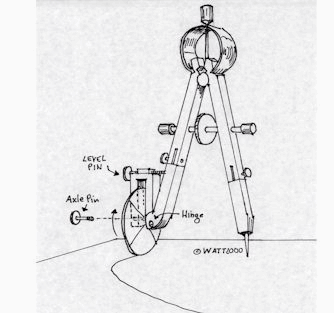"At [top] is a drawing of such an attachment which I call the "Z Compass.  The Z wheel is placed on the curve at some intersection on the curve (or straight line) and is then rolled to the next point for marking. The rolling is the curvilinear equivalent to opening a rectilinear compass's legs. One can now transfer some curvilinear distance to another point in the construction. This is not using a pre-existing "marked ruler", nor is it a "mechanical devise", any more than a compass is.   If it is permissible to use a 2 dimensional compass, why would it not be permissible to use a 3 dimensional compass, particularly given Euclidean geometry extensively examines both aspects of geometry? It is contrary to logic not to do so. The simplest version of this tool is a wheel pinned to a stick. The wheel is serving as a "3 dimensional compass". It is a tool based on my postulate substitution for Euclid's postulate 5 (see my geometry definitions at geometry definitions). The marks arrived at are from a "Rusty compass" (picking up the compass and moving it) format, which is allowed in Euclidean Geometry (i.e. Euclid's "Classic Solution" to drawing parallel lines).

"An example of its use; We draw a circle and find its hexagonal points. An unmarked "Z wheel" is placed in the leg and the compass legs are registered to the radius distance. A beginning mark is made on the wheel and the wheel is rolled along the circumference until it meets the first intersection of hexagonal leg and circumference. Another mark is made to notate the distance traveled along the curve by some portion of the Z wheel. That portion notated, is the exact curvilinear distance of the hexagonal arc transferred from the original circle to the Z wheel. The Z wheel can be moved to another section of the construction or the legs swung open to another radial proportion and the measure of the relative hexagonal arc of one circle transferred to a completely different arc. For the first time, one is directly measuring relative arcs in geometric construction and doing so without numbers, which is of primary concern in Euclidean geometry.

"It is a case of what is good for the goose is sauce for the gander. One cannot claim objectivity, allow rectilinear distance recording and then disallow curvilinear distance recording. To do so makes your study in logic irrelevant... "